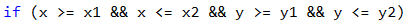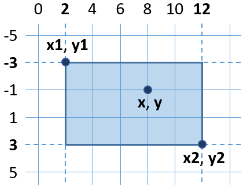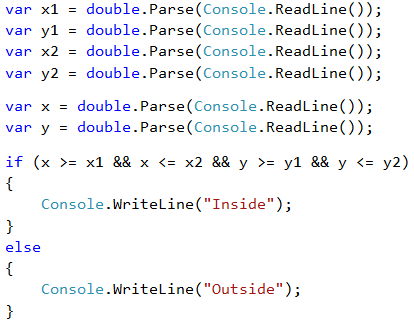# Logical "AND"

As we saw, in some tasks we have to make many checks at once. But what happens when in order to execute some code more conditions have to be executed and we don't want to make a negation (else) for each one of them? The option with nested if blocks is valid, but the code would look very unordered and for sure – hard to read and maintain.

The logical "AND" (operator &&) means a few conditions have to be fulfilled simultaneously. The following table of truthfulness is applicable:

a b a && b
true
true
false
false
true
false
true
false
true
false
false
false

## Video: Logical "AND"

Watch this video to learn how to use logical "AND" in programming: https://youtu.be/V86_z8GWarM.

## How the && Operator Works?

The && operator accepts a couple of Boolean (conditional) statements, which have a true or false value, and returns one bool statement as a result. Using it instead of a couple of nested if blocks, makes the code more readable, ordered and easy to maintain. But how does it work, when we put a few conditions one after another? As we saw above, the logical "AND" returns true, only when it accepts as arguments statements with value true. Respectively, when we have a sequence of arguments, the logical "AND" checks either until one of the arguments is over, or until it meets an argument with value false.

Example:

bool a = true;
bool b = true;
bool c = false;
bool d = true;
bool result = a && b && c && d;
// false (as d is not being checked)


The program will run in the following way: It starts the check form a, reads it and accepts that it has a true value, after which it checks b. After it has accepted that a and b return true, it checks the next argument. It gets to c and sees that the variable has a false value. After the program accepts that the argument c has a false value, it calculates the expression before c, independent of what the value of d is. That is why the evaluation of d is being skipped and the whole expression is calculated as false.## Example: Point in a Rectangle

Checks whether point {x, y} is placed inside the rectangle {x1, y1} – {x2, y2}. The input data is read from the console and consists of 6 lines: the decimal numbers x1, y1, x2, y2, x and y (as it is guaranteed that x1 < x2 and y1 < y2).

### Sample Input and Output

Input Output Visualization
2
-3
12
3
8
-1
Inside### Solution

A point is internal for a given polygon, if the following four conditions are applied at the same time:

• The point is placed to the right from the left side of the rectangle.
• The point is placed to the left from the right side of the rectangle.
• The point is placed downwards from the upper side of the rectangle.
• The point is placed upwards from the down side of the rectangle.